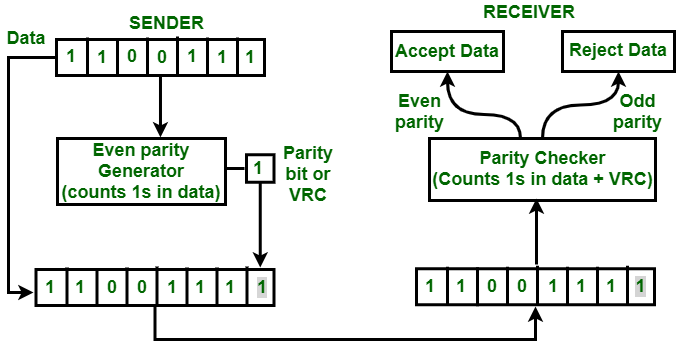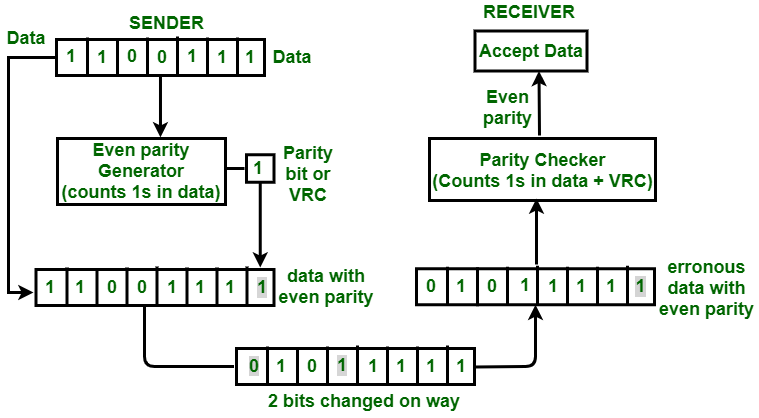Related Articles
Vertical Redundancy Check (VRC) or Parity Check
• Last Updated : 07 Sep, 2020

Vertical Redundancy Check is also known as Parity Check. In this method, a redundant bit also called parity bit is added to each data unit. This method includes even parity and odd parity. Even parity means the total number of 1s in data is to be even and odd parity means the total number of 1s in data is to be odd.

Example –
If the source wants to transmit data unit 1100111 using even parity to the destination. The source will have to pass through Even Parity Generator.Even parity VRC

Parity generator will count number of 1s in data unit and will add parity bit. In the above example, number of 1s in data unit is 5, parity generator appends a parity bit 1 to this data unit making the total number of 1s even i.e 6 which is clear from above figure.

Data along with parity bit is then transmitted across the network. In this case, 11001111 will be transmitted. At the destination, This data is passed to parity checker at the destination. The number of 1s in data is counted by parity checker.

If the number of 1s count out to be odd, e.g. 5 or 7 then destination will come to know that there is some error in the data. The receiver then rejects such an erroneous data unit.

• VRC can detect all single bit error.
• It can also detect burst errors but only in those cases where number of bits changed is odd, i.e. 1, 3, 5, 7, …….etc.